Courses

# Test: Linear Algebra - 4

## 20 Questions MCQ Test Topic-wise Tests & Solved Examples for IIT JAM Mathematics | Test: Linear Algebra - 4

Description
This mock test of Test: Linear Algebra - 4 for Mathematics helps you for every Mathematics entrance exam. This contains 20 Multiple Choice Questions for Mathematics Test: Linear Algebra - 4 (mcq) to study with solutions a complete question bank. The solved questions answers in this Test: Linear Algebra - 4 quiz give you a good mix of easy questions and tough questions. Mathematics students definitely take this Test: Linear Algebra - 4 exercise for a better result in the exam. You can find other Test: Linear Algebra - 4 extra questions, long questions & short questions for Mathematics on EduRev as well by searching above.
QUESTION: 1

Solution:
QUESTION: 2

Solution:
QUESTION: 3

### Let V be the space of twice differentiable functions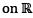satisfying f" - 2f' + f = 0. Define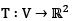by T(f') = (f'(0), f(0)), then T is

Solution:
QUESTION: 4

The determinant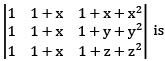Solution:
QUESTION: 5

Which of Ihe following matrices has the same row space as the matrix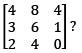Solution:
QUESTION: 6

The determinant of the n x n permutation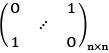[x] denotes greatest integer function of x.

Solution:
QUESTION: 7

The row space of a 20 x 50 matrix A has dimention 13. What is the dimension of the space of solution of Ax = 0?

Solution:
QUESTION: 8

Given a permutation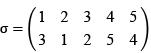the matrix A is defined to be the one whose ith column is the σ(i)th column of the Identity matrix I. Which of the following is correct?

Solution:
QUESTION: 9

For the matrix A as given below, which of them satisfy A6 = I?

Solution:
QUESTION: 10

Let I denote the 4 x 4 Identity matrix. If the roots of the characteristic polynomial of a 4 x 4 matrix M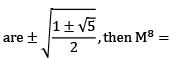Solution:
QUESTION: 11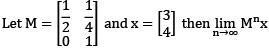Solution:
QUESTION: 12

Let M be the set of all invertibel 5 x 5 matrices with entries 0 and 1. For each m ∈ M, let n1 (m) and n0(m) denote the number of 1's and 0's in m respectively then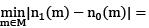Solution:
QUESTION: 13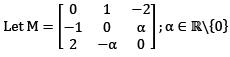and b a non zero vector such that Mx = b for some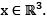Then the value of xTb is

Solution:
QUESTION: 14

The matrix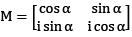is a unitary matrix when α is

Solution:
QUESTION: 15

Let P be the vector space over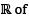all polynomials of degree less than 3 with real coefficients. Consider the linear transformation T : P → P defined by
T(a0 + a1x + a2x2 + a3x3) = a3 + a2x + a1x2 + a0x3

Then the matrix representation of M of T with respect to the ordered basis {1, x, x2,x3} satisfies

Solution:
QUESTION: 16

The largest eigenvalue of the matrix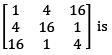Solution:
QUESTION: 17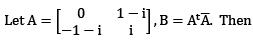Solution:
QUESTION: 18

Let a,b,c,d be distinct non zero real numbers with a + b = c + d. Then an eigenvalue of the matrix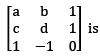Solution:
QUESTION: 19

The system of linear equations
x - y + 2z = b1
x + 2y - z = b2
2y - 2z = b3
is inconsistent when (b1, b2, b3) equals

Solution:
*Multiple options can be correct
QUESTION: 20

Let X and Y are n x n matrices with real entries, then which of the following is(are) true?

Solution: